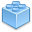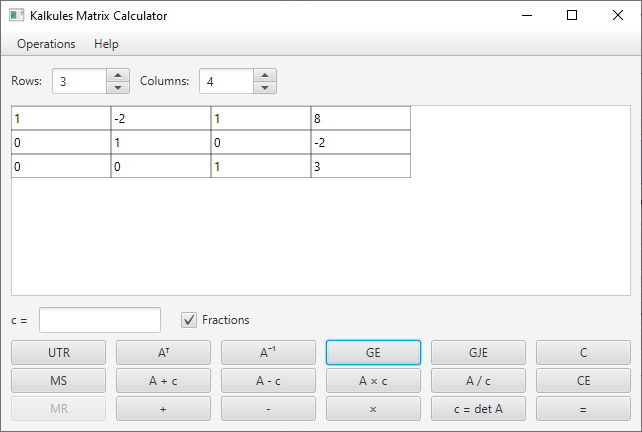# Kalkules Matrix Calculator

0.3.0
Kalkules (Freeware)
Free matrix calculator with a variety of features.Free matrix calculator
Free matrix calculator with a variety of features. Features: - Matrix addition, subtraction and multiplication. - Adding, subtracting, multiplying and dividing matrices by constants (scalar values) - Elementary row operations: - Switching 2 rows - Multiplying row by constant - Adding row multiplied by constant to another row - Elementary column operations: - Switching 2 columns - Multiplying column by constant - Adding column multiplied by constant to another column - Upper triangular matrix calculation - Transposed matrix calculation - Inverted matrix calculation - Gaussian elimination - Gauss-Jordan elimination - Determinant calculation - Matrix generation - Calculating with real numbers or fractions
Technical details
Title:
Kalkules Matrix Calculator 0.3.0 for Windows
Requirements:
none
OS Support:
Win2000, WinXP, Win7 x32, Win7 x64, Windows 8, Windows 10, WinServer, WinOther, WinVista, WinVista x64
Language:
English